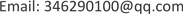ASSAdvances in Social Sciences2169-2556Scientific Research Publishing10.12677/ASS.2019.81011ASS-28495ASS20190100000_75687274.pdf人文社科 基于时间序列分析的云南省人口数量模型 Population Quantity Model of Yunnan Province Based on Time Series Analysis 健颖1*云南财经大学，统计与数学学院，云南 昆明1001201908016974© Copyright 2014 by authors and Scientific Research Publishing Inc. 2014This work is licensed under the Creative Commons Attribution International License (CC BY). http://creativecommons.org/licenses/by/4.0/Copyright © 2019 by author(s) and Hans Publishers Inc.

This work is licensed under the Creative Commons Attribution International License (CC BY).

http://creativecommons.org/licenses/by/4.0/1. 引言

2. 文献综述

3. 理论知识3.1. 趋势拟合法——线性模型 [<xref ref-type="bibr" rid="hanspub.28495-ref8">8</xref>] <sup> </sup>

{ x t = a + b t + I t E ( I t ) = 0 , V a r ( I t ) = δ 2

3.2. ARIMA模型 [<xref ref-type="bibr" rid="hanspub.28495-ref9">9</xref>] <sup> </sup>

{ Φ ( B ) ∇ d x t = Θ ( B ) ε t E ( ε t ) = 0 , V a r ( ε t ) = δ ε 2 , E ( ε t ε s ) = 0 , s ≠ t E ( x s ε t ) = 0 , ∀ s ≠ t

3.3. 精确度评价

1) 均方误差(RMSE)：

R M S E = ( 1 n ∑ i = 1 n ( x i − x i ^ ) 2 ) 1 / 2

2) 平均绝对百分比误差(MAPE)：

M A P E = 1 n ∑ i = 1 n | x i − x i ^ x i | × 100 %

4. 模型构建

4.1. 趋势拟合法——线性模型

1) 模型拟合

x = 2820.9909 + 47.5129 t (1)

F = 3396 ， R 2 = 0.9872

2) 模型检验

3) 精度评价

R M S E = 69.44 ， M A P E = 1.46 %

4.2. ARIMA模型 [<xref ref-type="bibr" rid="hanspub.28495-ref8">8</xref>] <sup> </sup>

1) 数据预处理

2) 模型拟合

x t = 0.0731 x t − 1 + ε t − 0.5731 ε t − 1 (2)

3) 模型检验

4)精度评价

R M S E = 8.62 ， M A P E = 0.15 %

4.3. 模型比较

Precision comparisons of Model (1) and Model (2
RMSEMAPE

69.448.621.46%0.15%

5. 模型预测

x t = 0.0731 x t − 1 + ε t − 0.5731 ε t − 1 (2)

Population forecast value table of Yunnan Province in the next five year

20184828.7(4811.447, 4845.958)
20194857.38(4826.277, 4888.49)
20204886.06(4840.07, 4932.055)
20214914.74(4852.573, 4976.91)
20224943.42(4863.782, 5023.059)

6. 结论